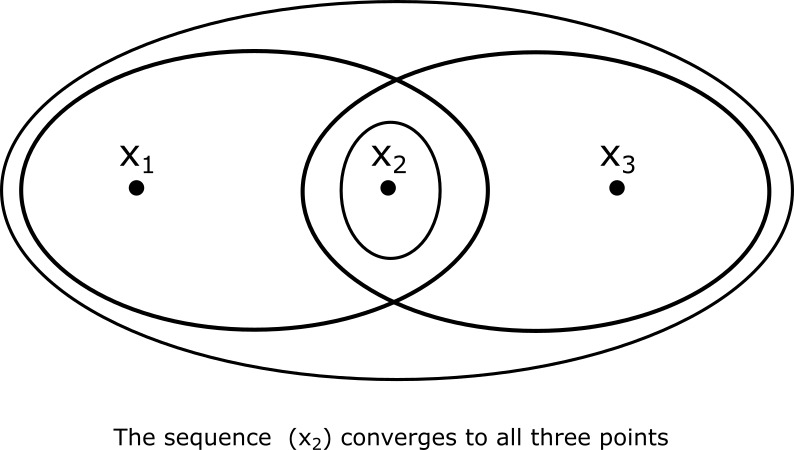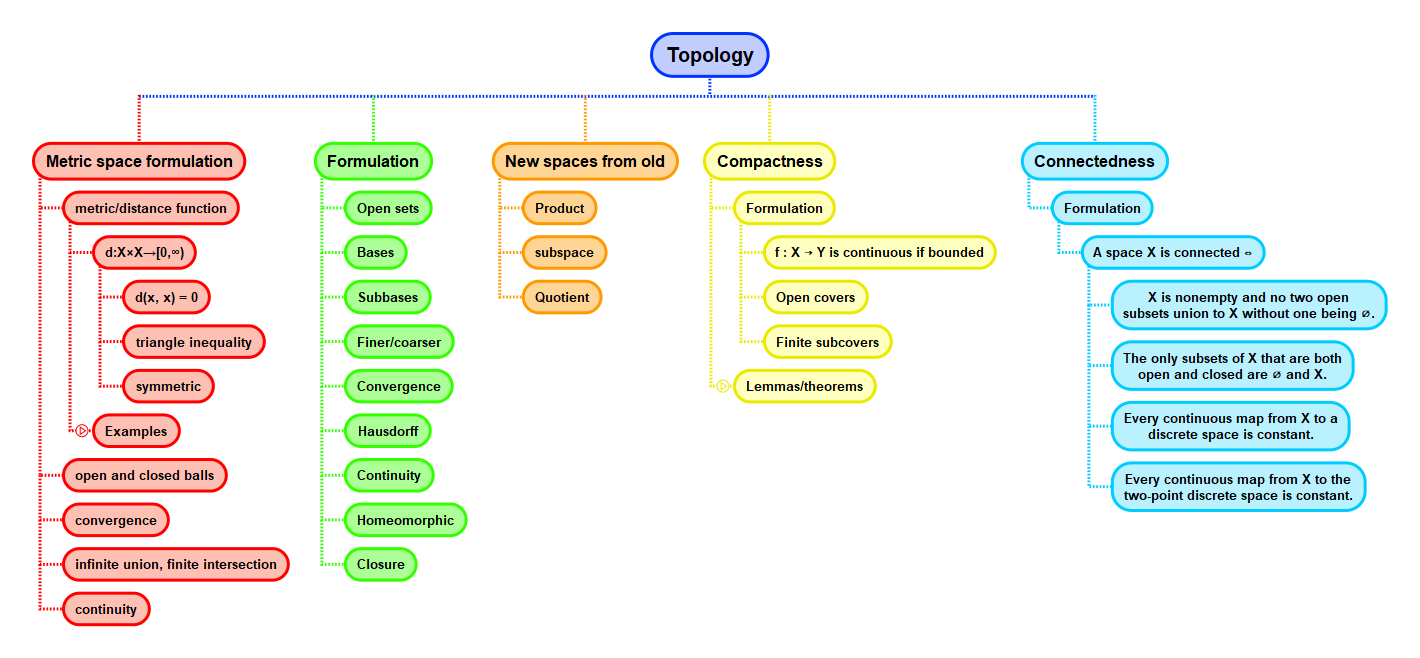Show Question
Math and science::Topology

# Sequence convergence

### Sequence convergence

Let $$X$$ be a topological space, let $$(x_n)$$ be a sequence in $$X$$ and let $$x_0 \in X$$. Then $$(x_n)$$ converges to $$x_0$$ iff for every neighbourhood $$U$$ of $$x_0$$ there is an $$N \ge 1$$ such that for every $$n \ge N$$, $$x_n \in U$$.

In other words, every neighbourhood of the point to which a sequence converges must contain the whole tail end of the sequences starting from some arbitrary point in the sequence.

It is a good exercise to check that this definition carries the expected meaning for metric spaces.

### Example

#### Sequences in Hausdorff spaces

A common demonstration of the importance of Hausdorff spaces:

Let $$X$$ be a Hausdorff topological space. Then each sequence in $$X$$ converges to at most one point.

Another good proof exercise.

#### Multiple convergence

An example where we get multiple convergence:## Context